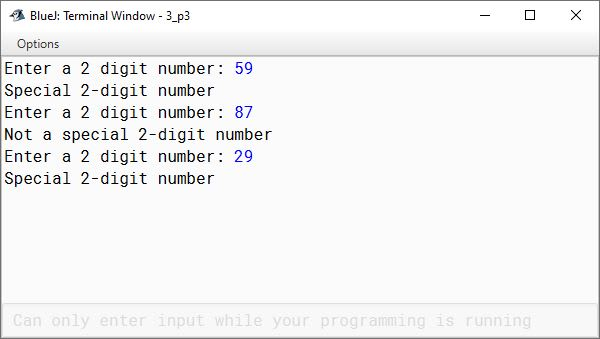# Solved Example: Special Number Java Program

## ICSE Computer Applications

In this lesson, we will look at a Java program which checks if a 2-digit number is a special number or not. This question came in 2014 board exam. Here is the question as it came in the exam:

A special two-digit number is such that when the sum of its digits is added to the product of its digits, the result is equal to the original two-digit number.

Example:
Consider the number 59.
Sum of digits = 5 + 9 = 14
Product of its digits = 5 x 9 = 45
Sum of sum of digits and product of digits = 14 + 45 = 59

Write a program to accept a two-digit number. Add the sum of its digits to the product of its digits. If the value is equal to the number input, output the message “Special 2-digit number” otherwise, output the message “Not a special 2-digit number”.

To check if a number is a special number or not, we will follow the below steps:

1. Extract the digits of the number.
2. Compute the sum of the digits.
3. Compute the product of the digits.
4. Add the sum and product of digits.
5. Check if the result from step 4 above is equal to the original number.

Here is our special number Java program:

```import java.util.Scanner;

public class KboatSpecialNumber
{
public void checkNumber() {

Scanner in = new Scanner(System.in);

System.out.print("Enter a 2 digit number: ");
int orgNum = in.nextInt();

int num = orgNum;

int digit1 = num % 10;
num /= 10;

/*
* If num becomes 0 after extracting first digit
* it means that user entered a single digit number.
* We should check for such invalid inputs.
*/
if (num == 0) {
System.out.println("Invalid input. Entered number is not a 2 digit number");
System.exit(0);
}

int digit2 = num % 10;
num /= 10;

/*
* If num does not become 0 after extracting second digit
* it means that entered number has 3 or more digits.
* We should check for such invalid inputs.
*/
if (num != 0) {
System.out.println("Invalid input. Entered number is not a 2 digit number");
System.exit(0);
}

int digitSum = digit1 + digit2;
int digitProduct = digit1 * digit2;

if ((digitSum + digitProduct) == orgNum)
System.out.println("Special 2-digit number");
else
System.out.println("Not a special 2-digit number");

}
}```

We first accept the number from the user with the help of scanner class and store it in `orgNum` int variable. As we will truncate the number, it is good to make a copy of it to preserve the original input. We will need the original number later in the program.

```int digit1 = num % 10;
num /= 10;

/*
* If num becomes 0 after extracting first digit
* it means that user entered a single digit number.
* We should check for such invalid inputs.
*/
if (num == 0) {
System.out.println("Invalid input. Entered number is not a 2 digit number");
System.exit(0);
}

int digit2 = num % 10;
num /= 10;

/*
* If num does not become 0 after extracting second digit
* it means that entered number has 3 or more digits.
* We should check for such invalid inputs.
*/
if (num != 0) {
System.out.println("Invalid input. Entered number is not a 2 digit number");
System.exit(0);
}```

In the above lines of the program, we extract the 2 digits of the number and store them in `digit1` and `digit2` int variables. Notice the 2 if checks, they validate that the number entered by the user is a 2-digit number. If after extracting the first digit, the number becomes zero, it means that the number user entered was a single digit number.

This line `System.exit(0)` is a new thing here that we haven’t talked about so far. exit is a function of System class, it is used to terminate the execution of the program. We often come across scenarios like these where it is not possible to continue with the execution of the program. In such situations, we make use of System.exit function to terminate the program. A call to System.exit function will terminate the JVM which is executing our program. We pass 0 as the argument to System.exit to indicate normal termination. Any non-zero argument indicates abnormal termination.

Moving on to the next if check, if after extracting 2 digits the number doesn’t become zero, it means that the number entered by the user has more than 2 digits. Here again, we are terminating the program using System.exit.

```int digitSum = digit1 + digit2;
int digitProduct = digit1 * digit2;

if ((digitSum + digitProduct) == orgNum)
System.out.println("Special 2-digit number");
else
System.out.println("Not a special 2-digit number");```

After extracting the digits the logic is straightforward. In fact, the question itself describes the solution in detail providing step-by-step instructions. You can’t ask for an easier examination question than this. We compute the sum and product of the digits, add them together and check if it is equal to the original number and display an appropriate message to the user.

So, if you just know how to extract the digits of a number, the question itself provides the rest of the solution. Such questions are easy scoring opportunities for you. With a little preparation you can score full marks in such questions.

Let's conclude this lesson by examining the output of this program.